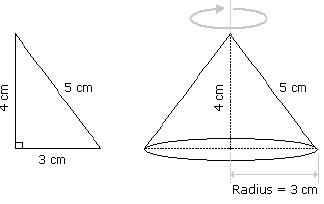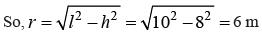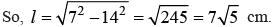Courses

Olympiad Test: Elementary Mensuration-II - 1

20 Questions MCQ Test Mathematics Olympiad Class 7 | Olympiad Test: Elementary Mensuration-II - 1

Description
Attempt Olympiad Test: Elementary Mensuration-II - 1 | 20 questions in 40 minutes | Mock test for Class 7 preparation | Free important questions MCQ to study Mathematics Olympiad Class 7 for Class 7 Exam | Download free PDF with solutions
QUESTION: 1

A right triangle with sides 3 cm, 4 cm and 5 cm is rotated the side of 3 cm to form a cone. The volume of the cone so formed is:

Solution:Clearly, we have r = 3 cm and h = 4 cm.
∴ Volume = 1/3 πr2h
= (1/3 × π × 32 × 4) cm3
= 12π cm3

QUESTION: 2

In a shower, 5 cm of rain falls. The volume of water that falls on 1.5 hectares of ground is:

Solution:

1 hectare = 10,000 m2
So, Area = (1.5 × 10000) m2 = 15000 m2
Depth     = (5/100) m = (1/20) m
∴ Volume = (Area × Depth)
= (15000 × 1/20) m3 = 750 m3

QUESTION: 3

A hall is 15 m long and 12 m broad. If the sum of the areas of the floor and the ceiling is equal to the sum of the areas of four walls, the volume of the hall is:

Solution:

Given 2(15 + 12) × h = 2(15 × 12)
⇒ h = (180/27) m = (20/3) m
∴ Volume = (15 × 12 × 20/3) m3
= 1200 m3

QUESTION: 4

66 cubic centimetres of silver is drawn into a wire 1 mm in diameter. The length of the wire in metres will be:

Solution:

Let the length of the wire be h.
Radius = 1/2 mm = 1/20 cm. Then,
⇒ (22/7 × 1/20 × 1/20 × h) = 66
∴ h = (60 × 20 × 20 × 7)/22 = 8400
cm = 84 m

QUESTION: 5

A hollow iron pipe is 21 cm long and its external diameter is 8 cm. If the thickness of the pipe is 1 cm and iron weighs 8 g/cm3, then the weight of the pipe is:

Solution:

Volume of iron
= (22/7 × [(4)2 – (3)2] × 21) cm3
= (22/7 × 7 × 1 × 21) cm3
= 462 cm3
∴ Weight of iron= (462 × 8) gm
= 3696 gm
= 3.696 kg

QUESTION: 6

A boat having a length 3 m and breadth 2 m is floating on a lake. The boat sinks by 1 cm when a man gets on it. The mass of the man is:

Solution:

Volume of water displaced
= (3 × 2 × 0.01) m3
= 0.06 m3
∴ Mass of man = Volume of water displaced × Density of water
= (0.06 × 1000) kg
= 60 kg

QUESTION: 7

50 men took a dip in a water tank 40 m long and 20 m broad on a religious day. If the average displacement of water by a man is 4 m3, then the rise in the water level in the tank will be:

Solution:

Total volume of water displaced
= (4 × 50) m3 = 200 m3
∴ Rise in water level = [200/( 40 × 20)] m
= 0.25 m =25 cm

QUESTION: 8

The slant height of a right circular cone is 10 m and its height is 8 m. Find the area of its curved surface.

Solution:

l = 10 m
h = 8 m∴ Curved surface area = πrl
= (π × 6 × 10) m2 = 60 π m2

QUESTION: 9

A cistern 6m long and 4 m wide contains water up to a depth of 1 m 25 cm. The total area of the wet surface is:

Solution:

Area of the wet surface
= [2(lb + bh + lh) – lb]
= 2(bh + lh) + lb
= [2 (4 × 1.25 + 6 × 1.25) + 6 × 4] m2
= 49 m2

QUESTION: 10

A metallic sheet is of rectangular shape with dimensions 48 m x 36 m. From each of its corners, a square is cut off so as to make an open box. If the length of the square is 8 m, the volume of the box (in m3) is:

Solution:

Clearly, l = (48 – 16)m = 32 m,
b = (36 – 16)m = 20 m
h = 8 m.
∴ Volume of the box = (32 × 20 × 8) m3
= 5120 m3

QUESTION: 11

The curved surface area of a cylindrical pillar is 264 m2 and its volume is 924 m3. Find the ratio of its diameter to its height.

Solution:

(πr2h)/ (2πrh) = 924/264
r = [(924 /264) × 2] = 7 m and, 2πrh = 264
h = (264 × 7/22 × 1/2 × 1/7) = 6 m
∴ Required ratio = 2r/h =14/6 = 7 : 3

QUESTION: 12

A cistern of capacity 8000 litres measures externally 3.3 m by 2.6 m by 1.1 m and its walls are 5 cm thick. The thickness of the bottom is:

Solution:

Let the thickness of the bottom be x cm.
Then, [(330 – 10) × (260 – 10) × (110 × x)]
= 8000 × 1000
⇒ 320 × 250 × (110 – x) = 8000 × 1000
⇒ (110 – x) = (8000 × 1000)/ (320 × 250) = 100
x = 10 cm = 1 dm

QUESTION: 13

What is the total surface area of a right circular cone of height 14 cm and base radius 7 cm?

Solution:

h = 14 cm, r = 7 cm.∴ Total surface area = πrl + πr2
= (22/7 × 7 × 75 + 22/7 × 72) cm2
= [154(5 + 1)] cm2 = (154 × 3.236) cm2
= 498.35 cm2

QUESTION: 14

A large cube is formed from the material obtained by melting three smaller cubes of 3, 4 and 5 cm side. What is the ratio of the total surface areas of the smaller cubes and the large cube?

Solution:

Volume of the large cube = (33 + 43 + 53)
= 216 cm3
Let the edge of the large cube be a.
So, a3 = 216 a = 6 cm
∴ Required ratio
= [6 × (32 + 42 + 52)]/ (6 × 62
= 50/36 = 25 : 18

QUESTION: 15

How many bricks, each measuring 25 cm × 11.25 cm × 6 cm, will be needed to build a wall of 8 m × 6 m × 22.5 cm?

Solution:

Number of bricks = Volume of the wall/ Volume of 1 brick = (800 × 600 × 22.5)/ (25 × 11.25 × 6)
= 6400

QUESTION: 16

What is the volume of 32 metre high cylindrical tank?
I. The area of its base is 154 m2.
​II. The diameter of the base is 14 m.

Solution:

Given, height = 32 m
From I, the area of the base = 154 m2
∴ Volume = (Area of the base × Height)
= (154 × 32) m3
Thus, I alone gives the answer.
From II, the radius of the base = 7 m
∴ Volume = π r2h = (22/7 × 7 × 7 × 32) m3
= 4928 m3
Thus, II alone gives the answer.

QUESTION: 17

Is a given rectangular block a cube?
I. At least 2 faces of the rectangular block are squares.
II. The volume of the block is 64.

Solution:

From I, any two of l, b, h are equal.
From II, lbh = 64.
From I and II, the values of l, b, h may be (1, 1, 64), (2, 2, 16), (4, 4, 4).
Thus, the block may be a cube or cuboid.

QUESTION: 18

What is the capacity of a cylindrical tank?
I. Radius of the base is half of its height which is 28 metres.
II. Area of the base is 616 sq. metres and its height is 28 metres.

Solution:

From I, h = 28 m and r = 14.
∴ Capacity = π r2h, which can be obtained.
Thus, I alone gives the answer.
From II, π r2 = 616 m2 and h = 28 m
∴ Capacity = (π r2 × h) = (616 × 28) m3
Thus, II alone gives the answer.

QUESTION: 19

What is the height of a circular cone?
I. The area of that cone is equal to the area of a rectangle whose length is 33 cm.
II. The area of the base of that cone is 154 sq. cm.

Solution:

II gives the value of r.
But, in I, the breadth of rectangle is not given.
So, we cannot find the surface area of the cone.
Hence, the height of the cone cannot be determined.

QUESTION: 20

What is the volume of a cube?
I. The area of each face of the cube is 64 square metres.
II. The length of one side of the cube is 8 metres.

Solution:

Let each edge be a metre.
Then, I. a2 = 64
a = 8 m
Volume = (8 × 8 × 8) m3 = 512 m3
Thus, I alone gives the answer.
II. a = 8 m
Volume = (8 × 8 × 8) m3 = 512 m3
Thus, II alone gives the answer.Use Code STAYHOME200 and get INR 200 additional OFF Use Coupon Code

Track your progress, build streaks, highlight & save important lessons and more!

Similar ContentRelated tests